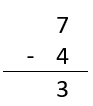Courses
Courses for Kids
Free study material
Free LIVE classes
More

# Subtraction by Crossing Out## Word Problems on Subtraction by Crossing Out

Subtraction is one of the four basic arithmetic operations you unknowingly use in your daily life. One of the many times you must have used subtraction in your daily life is when sharing toys or food with your sibling. In that case, how do you know the number of toys or other things you have left with you? In such cases, you perform subtraction. But how do you do it? Let’s learn an easy way to perform subtraction that is perfect for beginners: subtraction by crossing out.

## What is Subtraction by Crossing out?

Subtraction is performed when a quantity of items is taken away from the total items given. As discussed in the example where you have to give your brother/sister some toys or candy, you get the remaining candy by subtracting. Subtracting by crossing out is a simple way to learn subtraction. You arrange all the items and then cross out the number of items taken away from the total number of items.

## Examples of Subtraction by Crossing Out

Let’s continue with the same situation. Suppose you are the elder sibling, and your dad gives you 7 candies to eat and asks you to give 3 candies to your brother/sister. How many candies are left with you after giving 3 candies to your brother/sister?

We know that 3 candies are given to your sibling. Then, first, you put all seven candies in order and then cross three of them as shown in the subtraction picture given below.Crossing out candies

After crossing out, only four are left behind, as shown above. That means you have 4 candies left.

This subtraction can also be written as given below:

7 - 4 = 3

Or it is also written as given in the image belowTherefore, you also understand that to make 7, you have to add 3 to 4.

## Subtraction by Crossing Out Problems With Solution

Here are some subtraction word problems that you can easily solve by using the crossing-out method.

1. There are 3 balls with you. 2 of the balls are flat. How many balls can you play with?

Solution: The total number of balls is 3.

To find the number of balls perfect for playing, you can use the crossing-out subtraction method.Crossing out the balls

This way, you can see that there is only 1 ball left from the total of 3 balls.

So, the correct answer is 1.

We can also write it as \$3 - 2 = 1\$.

2. Suppose you have bought 4 ice creams. Of the 4 ice creams, 2 melted. How many ice creams are left with you?

Solution: There are four ice creams in total.

Two ice creams melted.

To find the number of remaining ice creams, you have to perform subtraction. By using the method of "crossing out," you can find the number of ice creams remaining.Crossing out ice-creams

As you can see from the above image, there are only two ice creams left.

We can also write it as, \$4 - 2 = 2\$.

So, the correct answer is 2.

3. Ravi’s father bought him 5 sweets and told him to give 2 sweets to his sister. How many sweets are left with Ravi?

Solution: The total no sweets that Ravi had = 5.

The number of sweets Ravi had to give her was 2.

Therefore, to find the number of sweets left with Ravi after giving them to her sister, you have to perform subtraction by crossing them out.Crossing out the given sweets

As a result, the remaining sweets after crossing out the ones given to his sister are three.

Therefore, the correct answer is 3.

This can also be written as, \$5 - 2 = 3\$.

## Conclusion

So far, you've learnt about subtraction and cross-out subtraction. You learnt that subtraction is used in daily life for various purposes. You have learnt that to find any remaining quantity, you can use subtraction. Subtraction by crossing out is nothing but the method where you cross out the images of the things that are subtracted from a total quantity. Apart from that, you have also learnt to solve word problems related to simple subtraction by using the crossing-out method.

Last updated date: 01st Oct 2023
Total views: 80.1k
Views today: 1.80k

## FAQs on Subtraction by Crossing Out

1. Can we use subtraction by the crossing out method for subtracting larger quantities?

The subtraction by crossing out method cannot be used to solve subtraction with large numbers or quantities like 100 or 200. It is perfect for kids who are just beginning to learn subtraction. Numbers like 10, 12, and 15 are fine for children to use the crossing-out method.

2. How to make subtraction look simple for kids?

To make subtraction look simple for kids, introduce them to the crossing-out method so that the child understands the meaning of subtraction from the beginning. These simple methods will help the child understand the fundamentals more quickly.

3. What are the other methods of subtraction apart from the crossing-out method?

Apart from crossing out, the other methods of subtraction are the part-whole method and the comparison method. These methods are also simple and easy to understand for children who are new to the concept of subtraction.